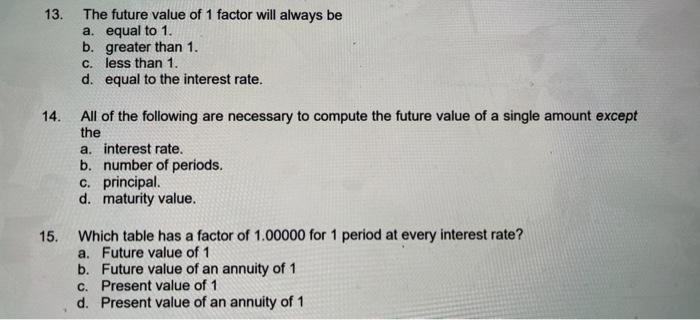# Question Solved1 Answerplease answer all parts of the wuestion for a big thumbs up and a good rating.Thank you. 13. The future value of 1 factor will always be a. equal to 1. b. greater than 1. c. less than 1. d. equal to the interest rate. 14. All of the following are necessary to compute the future value of a single amount except the a. interest rate. b. number of periods. c. principal d. maturity value. 15. Which table has a factor of 1.00000 for 1 period at every interest rate? a. Future value of 1 b. Future value of an annuity of 1 c. Present value of 1 d. Present value of an annuity of 1please answer all parts of the wuestion for a big thumbs up and a good rating.Thank you.
Transcribed Image Text: 13. The future value of 1 factor will always be a. equal to 1. b. greater than 1. c. less than 1. d. equal to the interest rate. 14. All of the following are necessary to compute the future value of a single amount except the a. interest rate. b. number of periods. c. principal d. maturity value. 15. Which table has a factor of 1.00000 for 1 period at every interest rate? a. Future value of 1 b. Future value of an annuity of 1 c. Present value of 1 d. Present value of an annuity of 1
More
Transcribed Image Text: 13. The future value of 1 factor will always be a. equal to 1. b. greater than 1. c. less than 1. d. equal to the interest rate. 14. All of the following are necessary to compute the future value of a single amount except the a. interest rate. b. number of periods. c. principal d. maturity value. 15. Which table has a factor of 1.00000 for 1 period at every interest rate? a. Future value of 1 b. Future value of an annuity of 1 c. Present value of 1 d. Present value of an annuity of 1